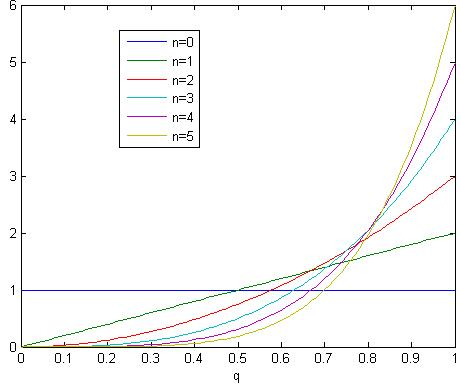# What is the probability that the sun will rise tomorrow?

Laplace introduced the sunrise problem in 18th century. With prior knowledge that the sun has risen N days in the past consecutively (not knowing the gravity rules etc), what is your confidence that the sun will rise tomorrow?

Let p be the probability that the sun rise. Apparently p range from 0 to 1. Without any prior knowledge, we have same level of confidence to believe p=0, 0.1, 0.2, …, or 1. So we assume equal probability distribution on p.

Now assume day 1 passed and we found the sun rose. Based on this knowledge, how should we update our belief on p? Well, p=0 is impossible, otherwise we will have not observed the sunrise. p=0.1 is possible but unlikely. Because if p=0.1 we only have 10% probability to observe a sunrise but we did observe one. We can actually formally calculate P(p=q|N=1) = P(N=1|p=q) * P(p=q) / P(N=1) using Bayes’s theorem. Since p(N=1) is in the denominator and it doesn’t depend on q, we don’t care. Our prior belief of P(p=q) was a uniform distribution. P(N=1|p=q) is equal to q. So the result is P(p=q|N=1) = q. After normalization (i.e. our total ‘belief’ over all p should be 1), P(p=q|N=1) = 2*q. So we have updated our belief from uniform distribution to a skewed distribution towards bigger probability of sunrise.

Based on our new belief (i.e. P(p=q)=2*q), what is our confidence that the sun will rise tomorrow? We simply need to sum (or integrate) all the possibilities. The final result equals to int(2*q^2) = 2/3. Yes, we have 2/3 confidence to say the sun will rise tomorrow, given we have observed one sunrise.

We then continue doing this for N=2, 3, …. The confidence level equals to (N+1)/(N+2). If we have observed sunrise for 1000 days, our confidence level raised to 0.999.The belief of the probability of sunrise based on n observations of sunrise

But if we have prior knowledge of the gravity rules, our belief will be dramatically different.## LaTex support (2020)

I used to use jsMath to support latex in this website, but it no longer works (I have no idea why). The one I am using right now in this blog is MathJax LaTex WordPress plugin. A pleasant surprise is that my old equations (e.g. in Balloon model) stil

When I was in high school and saw the following equation, my mind was blown! $$1+\frac{1}{4}+\frac{1}{9}+\frac{1}{16}+…=\frac{\pi^2}{6}$$ Why is Pi here? Isn’t it supposed to show up only in circle related problem? But the left-hand has n
1.Kate Kirby says: